﻿ Full-field Stress Analysis of a Crane Hook Model Performed by Finite Element Analysis and Digital Image Correlation MethodPublications are Open
Access in this journal
Article Versions
Export Article
• Normal Style
• MLA Style
• APA Style
• Chicago Style
Research Article
Open Access Peer-reviewed

### Full-field Stress Analysis of a Crane Hook Model Performed by Finite Element Analysis and Digital Image Correlation Method

Martin Hagara , Miroslav Pástor
American Journal of Mechanical Engineering. 2017, 5(6), 293-297. DOI: 10.12691/ajme-5-6-12
Published online: December 15, 2017

### Abstract

The contribution deals with a full-field stress analysis of the crane hook model. The investigation was realized using numerical as well as experimental analysis. In numerical analysis, the software based on finite element method was used. Experimental analysis was performed in laboratory conditions via non-contact optical method of digital image correlation. The results in a form of von Mises stress obtained by both approaches along identical virtual line gages, were depicted and compared. The paper briefly describes the methodologies of both, experimental and numerical types of modeling.

### 1. Introduction

The crane hooks are normalized components, made separately, however, serving as a part of robust machines such as cranes or excavators, whereby these machines are used for elevation and transmitting of heavy weights in industry or build grounds. The excavators with such hooks allow wider range of applications, because they are more moveable as cranes or autocranes and are able to perform excavation work as well as to ensure elevation and transmission of weights 1, 2.

There are several types of the hooks, whereby the simple or the double ones (see Figure 1) are used at most.

• Figure 1. Crane hook made with a latch: a simple (left), a double (right) 

Both of these types can include a latch. Also their attachment to the working machine can be made in different ways, e.g. through threaded joint, fixing between the crane grips or by pressing a shank to the hooks hole located at the end of the crane arm.

According to the dimensions described by a norm, the hooks are labeled by numbers from 006 for the smallest one up to 250 for the biggest one. They are also divided into five strength groups denoted by leters M, P, S, T and V due to the operation class of the crane, which they are attached to 4.

The crane hooks are made by technological process known as forging. Particular types of cranes can be forged without restraint (the smaller ones denoted by 006 up to 40) or in a die (the bigger ones denoted by 10 up to 250).

### 2. Analytical Solution of the Crane Hooks

From the analytical point of view the crane hooks can be classified as structures, well-known as very curved beams. The structures belong to the group of curved beams if fulfill the condition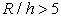, where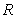is the radius of curvature and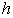is the height of the section.

By an analysis of the stress distribution across the section height, it is possible to determine the stress, caused by normal and tangential force, in the same way as by plain beams. However, the stress distribution, caused by bending differs from the stresses obtained in plain or small curved beams. To determine the relation for normal stress caused by bending it is necessary to consider the following conditions:

• the problem is solved only in elastic domain and the Young modulus has the same level for tension as well as compression loading,

• the Navier-Bernoulli hypothesis is relevant,

• the very curved beams have the plane of symmetry,

• the longitudinal fibers do not compress each other,

At chosen element of the very curved beam, loaded by moment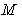, the coordinate system is oriented in such a way, that y-axis is coincident with the neutral axis of the section. The radius of curvature of the neutral axis is labeled as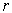. Momentis in equilibrium with the bending moment, which is a result of normal stresses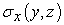acting in the section. The statics equation of equilibrium of the element depicted in Figure 2, can be expressed by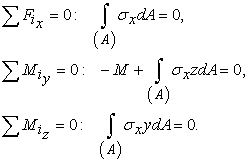(1)
• Figure 2. Very curved beam element

For determination of normal stress distribution depending on the bending moment, it is necessary to use the deformation condition for element depicted in Figure 3 5.

• Figure 3. Deformation of very curved beam element caused by moment M

The strain of the fiber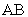, located in distance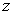from the neutral axis, can be expressed by relation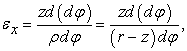(2)

from which due to Hook’s law for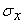is valid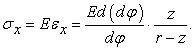(3)

From the moment equation of equilibrium to the y-axis it can be written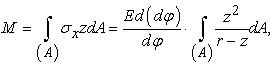(4)

that gives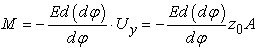(5)

where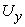is the static moment of the section area and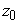is the negative value of distance of the neutral axis to the axis passing the center of mass.

Finally, normal stresscan be expressed by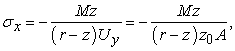(6)

what means, thatis changing along the height of the section due to the equation of hyperbola.

If the radiuses of the curvature of internal and external fibers are denoted as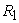and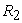, then the corresponding normal stresses for internal and external fibers are given by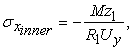(7)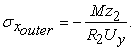(8)

If the radius of curvature of neutral axisis given by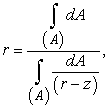(9)

then e.g. for the rectangular section with width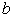and heightit is valid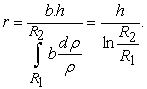(10)

The strength conditions valid for very curved beam loaded by a combination of tension and bending is given by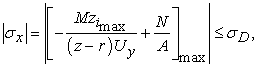(11)

where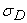is the design stress. The distribution of particular stress components (caused by tension (σt) and bending (σb)) across the height of section is depicted in Figure 4.

• Figure 4. Stress components distribution along the height of hook section 

### 3. The Numerical Stress Analysis of the Crane Hook

The numerical crane hook model was created due to STN 27 1902 norm 6. The dimensions (see Figure 5) and rectangular shape of its section were adapted to the purpose to perform an experimental stress analysis in laboratory conditions of authors’ workplace. For that reason, a straight-through hole with diameter of 8 mm was created in the upper part of hook serving for its loading. Thickness of the model was 10.2 mm that corresponds to the thickness of polymer plate, which the hook model, used in experimental analysis, was made from. The material of mentioned plate has the following properties: Young modulus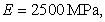Poisson ratio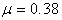and density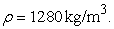• Figure 5. Shape and dimensions of the analyzed crane hook

On the created model, a static analysis was realized. Considering the boundary conditions (plane stress), the task was simplified to a 2D analysis. Because of that, the number of elements as well as nodes decreased rapidly, what meant the reduction of numerical analysis duration. The mesh, created by triangular elements, was in chosen locations of the model refined with element size of 1 mm (see Figure 6), other elements have the size of 4 mm.

• Figure 6. Mesh of triangular elements created in numerical analysis

Restraint was realized in a form of steel (AISI 1020) structure with circular character fixed around, located in bottom part of the hook. Between hook and mentioned structure, a contact called “No penetration”, was created. The crane hook model was loaded by tension force of 980 N.

In Figure 7 it is possible to see the full-field results of numerical analysis in a form of von Mises equivalent stress.

• Figure 7. Von Mises stress acquired on the surface of the analyzed hook via numerical analysis

### 4. The Experimental Stress Analysis of the Crane Hook

Experimental full-field stress analysis of the crane hook was realized via digital image correlation method. It is a non-contact method adherent to optical methods, where the information about the analyzed object in a form of displacements and strains, are determined through the comparison of digital images, known as correlation. The correlation is performed along small image elements, called facets. For that reason, the surface of the hook has to contain a random black and white speckle pattern.

The analysis was realized using correlation system Q-400 Dantec Dynamics containing two low-speed cameras with 5Mpx image resolution. The arrangement of the measuring chain together with high-power source of white light ensuring a uniform illumination of the analyzed object can be seen in Figure 8. Loading was realized using mechanical device, whereby the value of tension force was registered through gage RSCC/200kg, connected to the strain indicator Vishay P3.

The fields of view captured by both cameras are depicted in Figure 9.

• Figure 8. Measuring chain used for the realization of the experimental stress analysis via digital image correlation
• Figure 9. The fields of view acquired by both cameras of the correlation system Q-400 Dantec Dynamics

Due to the size of speckles, the facet size was set to 20 px. To increase the resolution of the results the facets were 5 px overlaped. The obtained results from Istra4D v.4.3.0, that is a control software for correlation systems Dantec Dynamics are in a form of displacement as well as strain fields. To ensure a comparison of the results in a form of equivalent stress, the acquired data were imported and processed in Matlab. Von Misses equivalent stress was calculated in the center of each facet due to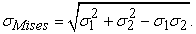(12)

Von Mises stress field depicted in Figure 10 introduces the results obtained experimentally through digital image correlation system Q-400 Dantec Dynamics. As can be seen the stress distribution in the area of interest seems to be equivalent to the stress distribution acquired numerically.

For the purpose of quantitative comparison of the obtained results, a virtual gage in a form of line was chosen. The location of this gage was coincident with the horizontal dot-and-dashed line depicted in Figure 5. It has to be stated that the edges of hook contour determined by digital image correlation method do not exactly coincide with the edges of hook numerical model. This fact is caused due to the methodology of stereoscopic digital image correlation and therefore the length and the locations of a starting point as well as an ending point of the line gage are not equal. Considering mentioned feature Figure 11 shows the comparison of the von Mises stress obtained on the identical parts of line virtual gages.

• Figure 10. Von Mises stress acquired on the area of interest of the analyzed hook via experimental analysis

As can be seen there is a good conjunction between the results obtained in first 30% of the virtual line gage length, where the relative difference reaches the values from the range of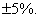The biggest difference occurs in the relative position range from 40% to 60%, where the relative difference reaches up to 30%. This phenomenon can be caused by the limitations of the cameras that’s resolution allows to measure strain up to 10-4 with sufficient accuracy. The values of relative difference increase in the last part of the virtual line gage.

• Figure 11. Von Mises stress acquired on the identical virtual line gage via numerical as well as experimental analysis

The marked difference described above can also be caused by the fact that by experimental investigation it is not easy to ensure the same boundary conditions as in numerical analysis. Therefore, an analysis was performed, which serves to predict what happens if the loading force deflect from the vertical direction about 1°, 2° or 3° (see the coarses depicted in Figure 12).

• Figure 12. The change of von Mises stress distribution after deflection of loading force from vertical direction

### 5. Summary

The paper describes the possibilities of performing full-field stress analysis on the surface of crane hook using numerical (finite element analysis) as well as experimental (digital image correlation) approach.

From the comparison of the results obtained experimentally and numerically, it was determined, that the stress obtained in the area of stress concentrator differs maximally in ca. ±5%. The biggest difference was achieved in the area of the hook neutral axis, where the possibilities of digital image correlation does not allow to get results with sufficient accuracy. The authors also notice to relatively big differences obtained in the results after small changes in the boundary conditions.

### Acknowledgements

The paper is the result of the project implementation VEGA 1/0751/16 and ITMS:26220120060 supported by the Research & Development Operational Programme funded by the ERDF.

### References

  Chetan, N. B. and Wankhade, N. A., Finite Element Stress Analysis of Crane Hook with Different Cross Sections, International Journal For Technological Research In Engineering, 1(9). 868-872, 2014. In article View Article  Chetan, N. B. and Wankhade, N. A., Design and Analysis of Crane Hook – Review, International Journal of Engineering Research & Technology, 3(1). 2818-2822, 2014. In article  Eld: PRODUKTY: Haki WG DIN [online]. Available on: http://www.eld.com.pl/pl/,produkty, 42. In article View Article  Vingu: Kované háky dle DIN 15401 / 15402 [online]. Available on: http://www.vingu.cz/katalog/kovane-haky-dle-din-15401-15402. In article View Article  Trebuňa, F. and Šimčák, F.: Odolnosť prvkov mechanických sústav. Košice: EMILENA, 2004. In article  STN 27 1902. 1963. Žeriavy a zdvíhadlá. Jednoduché kované háky s driekom. Tvar a rozmery výkovkov. In articleThis work is licensed under a Creative Commons Attribution 4.0 International License. To view a copy of this license, visit http://creativecommons.org/licenses/by/4.0/